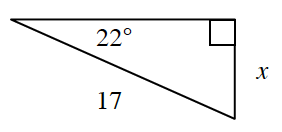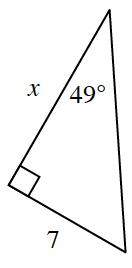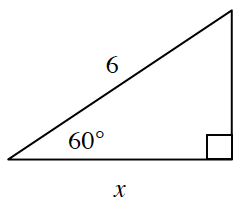### Home > INT2 > Chapter 4 > Lesson 4.2.2 > Problem4-72

4-72.

For each triangle below, write an equation relating the reference angle (the given acute angle) with the two side lengths of the right triangle. Then solve your equation for $x$.

Recall:
$\array{\sin\theta=\frac{\text{opp}.}{\text{hyp}.}\\ \cos\theta=\frac{\text{adj}.}{\text{hyp}.}\\ \tan\theta=\frac{\text{opp}.}{\text{adj}.}}$

1.1.1.$\cos\left(60^{\circ}\right)=\frac{x}{6}$

1. For part (c), use the complement of the reference angle to write a different equation. Solve for $x$ to confirm your answer for part (c).